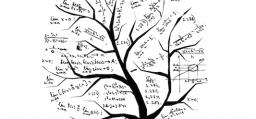# Equation 4055

Solve the equation and perform the test:
a) 3 (5-2x) + 5x = 5 -3 (x -1)
b) 2 (4y + 3) -3 = 2 - 5 (1 - y)

x =  -3.5
y =  -2

### Step-by-step explanation:

3(5-2x) + 5x = 5 -3 (x -1)

3·(5-2·x) + 5·x = 5 -3·(x -1)

2x = -7

x = -7/2 = -3.5

x = -7/2 = -3.5

Our simple equation calculator calculates it.

2(4y + 3) -3 = 2 - 5 (1 - y)

2·(4·y + 3) -3 = 2 - 5·(1 - y)

3y = -6

y = -6/3 = -2

y = -2

Our simple equation calculator calculates it.Did you find an error or inaccuracy? Feel free to write us. Thank you!

Tips for related online calculators
Do you have a linear equation or system of equations and looking for its solution? Or do you have a quadratic equation?